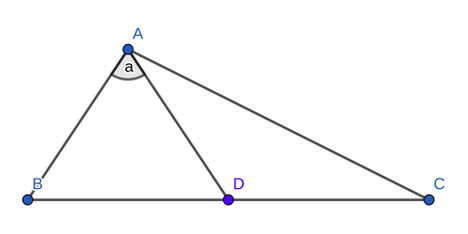# Piece of CakeNational Girls' Programmi...
Limits 1s, 512 MBDiya has got a nice birthday cake for Arshiya and Alayna, her twin daughters. The two sisters were amazed to see the strange triangular-shaped birthday cake. On the cake ABC, there is a line from one of the vertex A to the midpoint of the opposite line BC. On her daughter's birthday, Diya declared a quiz competition. She told her daughters that she will make two more square-shaped cake as the prize of that quiz competition. Each side length of Arshiya's cake will be equal to AB, one side of the triangle-shaped cake. Each side length of Alayna's cake will be equal to AC, one side of the triangle-shaped cake. For getting the prize, Arshiya and Alayna have to calculate the sum of the area of these two square-shaped cake. As they want to win the prize, they want your help.
You will be given some information about the triangle-shaped cake for solving the challenge. You will be given AB, the one side of the triangular-shaped cake, AD, the line from vertex A to the midpoint of BC and the angle DAB.

Consider $\pi = cos^{-1}(-1.0)$ if needed.

## Input

First line of the input contains an integer T(1 < T < 100000) which denotes the number of test cases. Then for each test case, there will be three real number X which denotes the length of AB, Y which denotes the length of AD and A which denotes the angle DAB. All lengths are less than 104, also angles and lengths are greater than zero.

It is guaranteed that the input forms a valid triangle with positive area.

## Output

For each test case, output the total sum of the area of the two square-shaped cake. Absolute error less than 10-6 will be ignored.

## Sample

InputOutput
2
100 86.60254037844386 30
30.341180 50.312433 52.911429
20000.000000000
8284.228534779### Submit